# Teach Tough Concepts: Closed-Loop Control with LabVIEW and a DC Motor

Updated Apr 11, 2023

## Environment

### Hardware

• NI ELVIS
• Quanser QNET DC Motor Control for NI ELVIS

### Software

• LabVIEW
• LabVIEW Control Design and Simulation Module
• LabVIEW MathScript Module
The concepts of control are essential for understanding natural and man-made systems. Since control is a systems field, to get a full appreciation of control it is necessary to cover both theory and applications. The skill base required in control includes modeling, control design, simulation, implementation, tuning, and operation of a control system. This tutorial shows how these concepts can be taught to students through use of a Quanser DC Motor plug-in board for the NI ELVIS, and LabVIEW Control Design and Simulation with LabVIEW MathScript RT. Traditionally, tuning a controller requires multiple iterations and trial and error to perfect. However, LabVIEW allows you to tune your controller in real time and then move directly into verification with a seamless integration with hardware.

By the end of this tutorial you will be able to:
• Model a DC motor
• Design a closed-loop PI controller
• Tune the controller in simulation
• Implement your controller with DC motor

### Step 1 - Modeling

The first step to designing a closed-loop controller is to identify a mathematical representation of the plant, or create a model. Many types of systems can modeled, including mechanical systems, electronic circuits, analog and digital filters, and thermal and fluid systems. For this experiment, we are going to create a model for a DC motor.

The DC motor can be best represented by a transfer function. A transfer function provides a mathematical description for how the inputs and outputs of a system are related. In our case, the input to the system is voltage (Vm) and the output from the system is angular velocity (Ωm). We can use the equation below to represent the model of our DC Motor where:

km = Motor back-EMF constant (V/(rad/s))
Rm = Motor armature resistance (Ohms)
Jeq = Equivalent moment of inertia (kg*m2) (Assume that Jeq=Jm(Motor armature moment of inertia))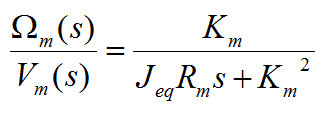Figure 1. Mathematical Model or Transfer Function for a DC Motor

This model will be used to design a closed-loop controller, which can then be tested with the actual motor. We can represent this transfer function in LabVIEW by using a MathScript Node which is part of the LabVIEW MathScript RT Module. The input parameter values were obtained from the Quanser QNET DC Motor specifications sheet.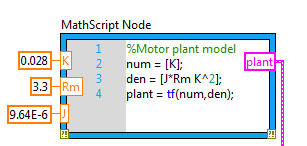Figure 2. Model of the DC Motor represented in the LabVIEW MathScript Node

The MathScript node can be found under the Programming >> Structures palette.

### Step 2 - Control Design

The next step is to choose a control method and design a controller. When designing a controller it is best to fully understand the plant, in our case, the DC Motor. This understanding comes from analysis of specialized graphs, such as Bode, root-locus, and Nyquist plot, which build intuition of how the plant will behave. Graphs in the time domain, such as the step response, provide immediate feedback on the ideal behavior of the system, such as rise time, overshoot, settling time, and steady-state error.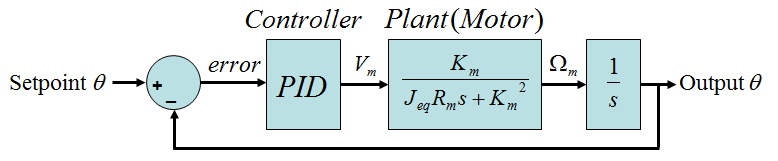Figure 3: Schematic of a Closed-Loop Control System

For this experiment we will design a PI controller for our DC motor using the LabVIEW Control Design and Simulation module. The Simulation Loop, which includes a built-in ODE solver for handling integrals and derivative terms, can be found in the Control Design and Simulation palette under Simulation. The Summation, Gain, Integrator and Transfer Function blocks can also be found in the Control Design and Simulation palette under Simulation >> Signal Arithmetic and Simulation >> Continuous Linear Systems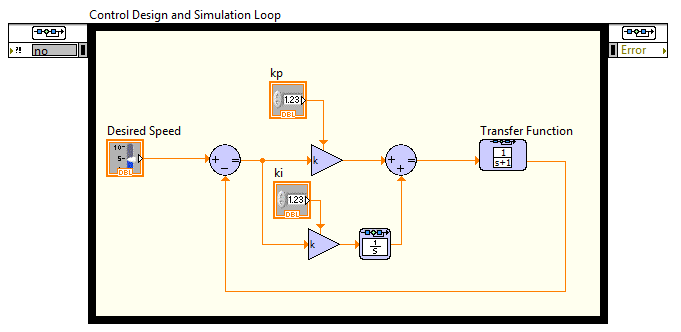Figure 4: Closed-Loop PI Controller in LabVIEW Control Design and Simulation

### Step 3 - Simulation

The next step is to simulate the response of the DC Motor when modifying the setpoint or desired speed input. This will allow us to tune the controller parameters or gains to increase the robustness of our system. We will need to combine the Transfer Function or model of the DC motor that we created in Step 1 with our closed-loop controller.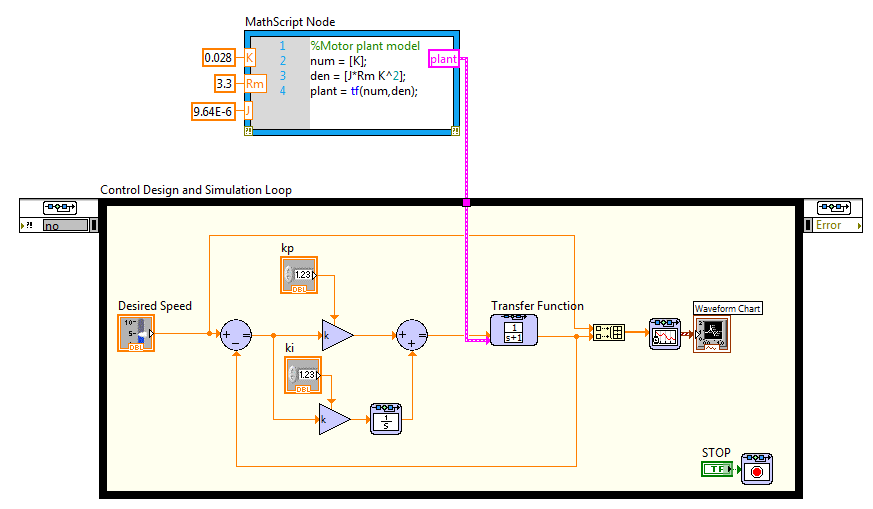Figure 5: Closed-Loop PI Controller with DC Motor Transfer Function

### Step 4 - Tuning and Verification

Now that we can simulate both our controller and the response of the DC motor, we can follow an iterative process to optimize our controller. We will tune the controller parameters from the LabVIEW front panel while verifying the system performance.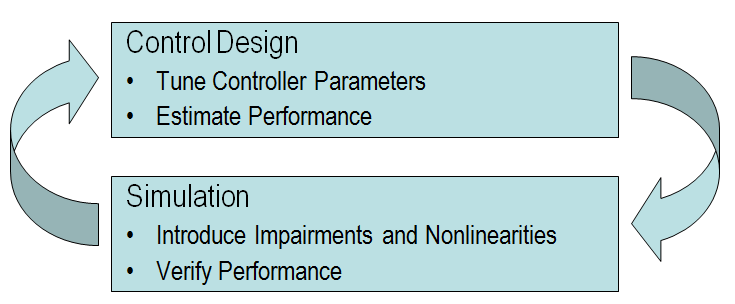Figure 6: An Iterative Process for Optimizing the Controller

We can use the following steps to tune our controller parameters:

• Begin with gains set at: Kp = 1 and Ki = 0
• Increase Proportional Gain (Kp) to get desired rise time
• Increase Integral Gain (Ki) to reduce steady-state error if necessary

Once we run the program, we can see the desired motor speed and the estimated motor speed plotted on the Waveform chart. While the rise time looks good with our proportional gain, kp set to 1, the plot shows a small amount of stead-state error, which is represented by the gap between the desired speed data and the estimated speed data. We can reduce this steady-state error by increasing our integral gain, ki.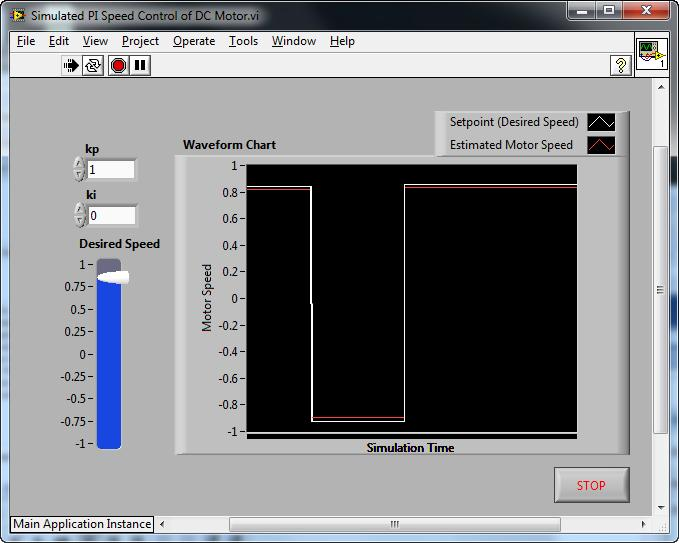Figure 7: Simulating the DC Motor Response with a Proportional (P) Controller

If we increase the integral gain, ki, to 10, we have a much better system response.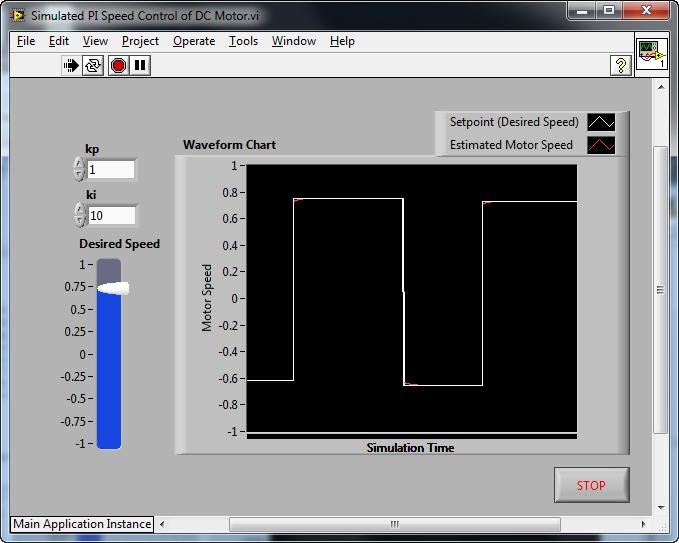Figure 8: Simulating the DC Motor Response with a Proportional-Integral (PI) Controller

### Step 5 - Implementation

Now that we've verified that our PI controller works with the simulated DC motor response, we can implement our finalized control system and control the speed of our Quanser DC Motor plug-in board for NI ELVIS. The LabVIEW Control Design and Simulation Module can be used to control real life systems as well as simulated models. To migrate from simulated control to real life control, the plant model can be replaced with hardware input and output functions.  In this case, we will replace the transfer function representing the DC motor with Data Acquisition (DAQ) input and output VIs that control the actual motor.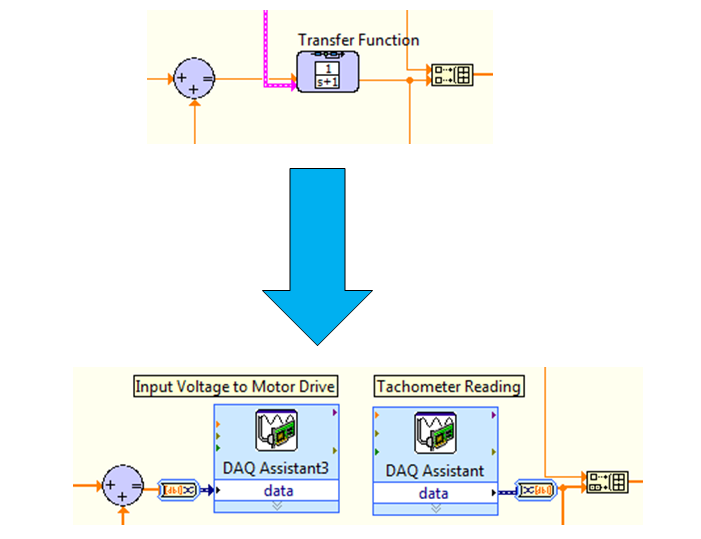Figure 9: Migrate from Simulation to Real Hardware by Replacing Transfer Function with Hardware Input/Output Blocks

Timing is an important consideration when using the LabVIEW Control Design and Simulation Loop with real hardware. Since the Control Design and Simulation Loop uses a built in ODE solver with time steps, it is important to set the Simulation Parameters and the Timing Parameters of the loop to have the same time step.  Data acquisition tasks typically use timing parameters, so it is also important to equate the Simulation loop timing with the data acquisition timing.

We can now run the LabVIEW program and control the speed of the Quanser DC Motor from the LabVIEW front panel.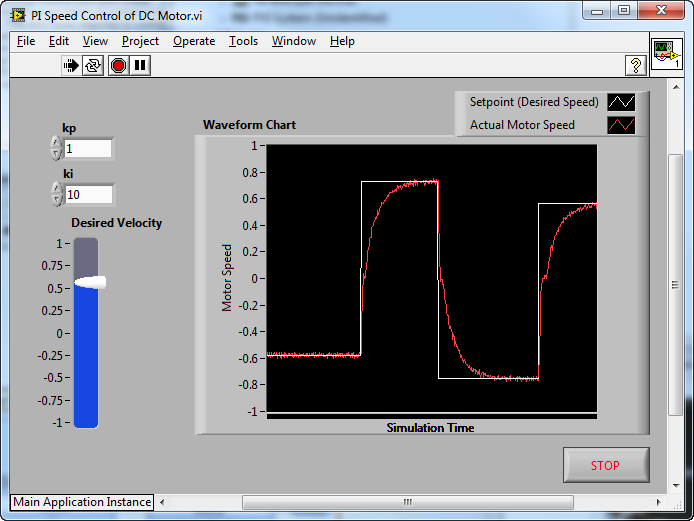Figure 10: Response of the Actual Quanser DC Motor with our PI Closed-Loop Controller

### 1. Solution Block Diagram VI Snippet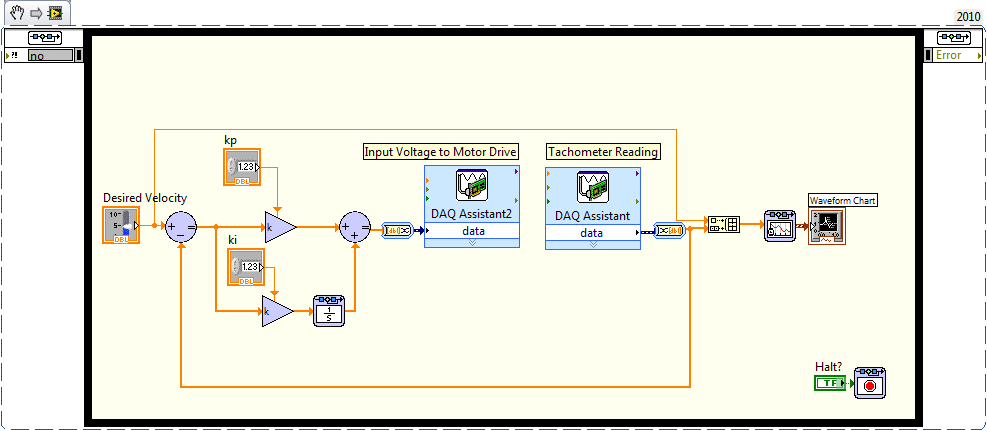Right-click on the above VI Snippet and select Save Image As...  Locate the file on your hard disk and click-and-drag the file icon onto your LabVIEW Block Diagram.  LabVIEW will automatically generate the code form the VI Snippet. Click here for more information on VI Snippets.